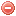# Description

The Drought code ($$DC$$) is one of the three fuel moisture code components of the Canadian forest fire weather index ($$FWI$$) system. The $$DC$$ represents the moisture content of deep compact layer of organic matter weighing about 25 kg/m2 when dry. It assesses the effects of seasonal drought on deep duff layers and heavy fuels. It requires noon temperature, precipitation and current month (in order to take daylength into account) as input data (Van Wagner 1987).

As the two other moisture codes of the $$FWI$$ (cf. $$FFMC$$ and $$DMC$$), the $$DC$$ comprises two phases: one for wetting by rain and one for drying.

# Formula

The $$DC$$ is calculated as follows (Van Wagner and Pickett 1985):

First, the previous day's $$DC$$\) becomes $$DC_{t-1}$$.

In case of rain (i.e. if $$P>2.8$$), the following procedure for wetting phases has to be chosen:

Effective rainfall $$P_d$$ [mm] is first calculated:

$P_d=0.83 \cdot P-1.27, \mbox{ for P}>2.8$

Then, the moisture equivalent of the previous day's $$DC$$, $$Q_{t-1}$$, has to be calculated:

$Q_{t-1}=800 \cdot e^{\frac{-DC_{t-1}}{400}}$

Based on that $$Q_{t-1}$$, the moisture equivalent after rain $$Q_{r_t}$$can be calculated:

$Q_{r_t}=Q_{t-1}+3.937\cdot P_d$

Finally, $$Q_{r_t}$$ can be converted to the the $$DC$$ after rain $$DC_{r_t}$$, which becomes the new $$D_{t-1}$$:

$DC_{r_t}=400\cdot\ln \Big(\frac{800}{Q_{r_t}}\Big), \mbox{ if }DC_{r_t}<0\mbox{, then } DC_{r_t}=0$

NB: if $$P\leqslant{2.8}$$, the above rainfall routine must be omitted.

Then, potential evapotranspiration $$V$$ has to be calculated as follows:

$V=0.36\cdot(T_{12}+2.8)+L_f, \mbox{ if }T_{12}<{-2.8}\mbox{, then } T_{12}={-2.8}$

where the day-length factor $$L_f$$ for each month is given in the following table:

Jan Feb Mar Apr May Jun Jul Aug Sep Oct Nov Dec
$$L_f$$ -1.6 -1.6 -1.6 0.9 3.8 5.8 6.4 5.0 2.4 0.4 -1.6 -1.6

NB: $$V$$ cannot be negative. If the above equation produces a negative result, then $$V$$ has to be set to 0.

Finally, $$DC$$ is calculated as follows:

$DC_t= \begin{cases} DC_{t-1}+0.5\cdot V, & \mbox{for }P\leqslant{2.8} \\ & \\ DC_{r_t}+0.5\cdot V, & \mbox{for }P>{2.8} \end{cases}$

The $$DC$$ is supposed to be calculated on a daily basis. The meteorological data used for its calculation have to be recorded at noon (for fire danger prediction at about 4 pm).

The $$DC$$ calculation starts, in regions normally covered by snow in winter, on the third day after snow has essentially left the area. In regions where snow cover is not a significant feature, the calculation starts on the third successive day with noon temperature greater than 12 °C (Lawson and Armitage 2008). The starting value of the index has to be set to 15.

Original publications:
Van Wagner and Pickett (1985)
Van Wagner (1987)

Other publication:
Lawson and Armitage (2008)

### Bibliography SearchNo results!

No results were found. Are you sure you searched for a tag?

### Symbols

 Variable Description Unit $$T$$ air temperature °C $$T_{dew}$$ dew point temperature °C $$H$$ air humidity % $$P$$ rainfall mm $$U$$ windspeed m/s $$w$$ days since last rain (or rain above threshold) d $$rr$$ days with consecutive rain d $$\Delta t$$ time increment d $$\Delta{e}$$ vapor pressure deficit kPa $$e_s$$ saturation vapor pressure kPa $$e_a$$ actual vapor pressure kPa $$p_{atm}$$ atmospheric pressure kPa $$PET$$ potential evapotranspiration mm/d $$r$$ soil water reserve mm $$r_s$$ surface water reserve mm $$EMC$$ equilibrium moisture content % $$DF$$ drought factor - $$N$$ daylight hours hr $$D$$ weighted 24-hr average moisture condition hr $$\omega$$ sunset hour angle rad $$\delta$$ solar declination rad $$\varphi$$ latitude rad $$Cc$$ cloud cover Okta $$J$$ day of the year (1..365/366) - $$I$$ heat index - $$R_n$$ net radiation MJ⋅m-2⋅d-1 $$R_a$$ daily extraterrestrial radiation MJ⋅m-2⋅d-1 $$R_s$$ solar radiation MJ⋅m-2⋅d-1 $$R_{so}$$ clear-sky solar radiation MJ⋅m-2⋅d-1 $$R_{ns}$$ net shortwave radiation MJ⋅m-2⋅d-1 $$R_{nl}$$ net longwave radiation MJ⋅m-2⋅d-1 $$\lambda$$ latent heat of vaporization MJ/kg $$z$$ elevation m a.s.l. $$d_r$$ inverse relative distance Earth-Sun - $$\alpha$$ albedo or canopy reflection coefficient - $$\Delta$$ slope of the saturation vapor pressure curve kPa/°C $$Cc$$ cloud cover eights $$ROS$$ rate of spread m/h $$RSF$$ rate of spread factor - $$WF$$ wind factor - $$WRF$$ water reserve factor - $$FH$$ false relative humidity - $$FAF$$ fuel availability factor - $$PC$$ phenological coefficient -

 Suffix Description $$-$$ mean / daily value $$_{max}$$ maximum value $$_{min}$$ minimum value $$_{12}$$ value at 12:00 $$_{13}$$ value at 13:00 $$_{15}$$ value at 15:00 $$_{m}$$ montly value $$_{y}$$ yearly value $$_{f/a}$$ value at fuel-atmosphere interface $$_{dur}$$ duration $$_{soil}$$ value at soil level

 Constant Description $$e$$ Euler's number $$\gamma$$ psychrometric constant $$G_{SC}$$ solar constant $$\sigma$$ Stefan-Bolzmann constant